# Introduction

Creel surveys allow fisheries scientists and managers to collect data on catch and harvest, an angler popuation (including effort expended), and, depending on survey design, biological data on fish populations. Though important methods of collecting data on the user base of the fishery, creel surveys are difficult to implement and, in graduate fisheries programs, creel surveys are paid little attention. As a result, fisheries managers–the first job for many fisheries-program graduates–often inherit old surveys or are told to institute new surveys with little knowledge of how to do so.

Fisheries can cover large spatial extents: large reservoirs, coast-lines, and river systems. A creel survey has to be statistically valid, adaptable to the geographic challenges of the fishery, and cost efficient. Limited budgets can prevent agencies from implementing creel surveys; the AnglerCreelSurveySimulation was designed to help managers explore the type of creel survey that is most appropriate for their fishery, including fisheries with multiple access points, access points that are more popular than others, variation in catch rate, the number of surveyors, and seasonal variation in day-lengths.

The AnglerCreelSurveySimulation package does require that users know something about their fishery and the human dimensions of that fishery. A prior knowledge includes mean trip length for a party (or individual), the mean catch rate of the

The AnglerCreelSurveySimulation package is simple, but powerful. Four functions provide the means for users to create a population of anglers, limit the length of the fishing day to any value, and provide a mean trip length for the population. Ultimately, the user only needs to know the final function ConductMultipleSurveys but because I’d rather this not be a black box of functions, this brief introduction will be a step-by-step process through the package.

## A walk-through of the package

This tutorial assumes that we have a very simple, small fishery with only one access point that, on any given day, is visited by 100 anglers. The fishing day length for our theoretical fishery is 12 hours (say, from 6 am to 6pm) and all anglers are required to have completed their trip by 6pm. Lastly, the mean trip length is known to be 3.5 hours.

For the purposes of this package, all times are functions of the fishing day. In other words, if a fishing day length is 12 hours (e.g., from 6 am to 6pm) and an angler starts their trip at 2 and ends at 4 that means that they started their trip at 8 am and ended at 10 am.

The make_anglers() function builds a population of anglers:


library(AnglerCreelSurveySimulation)

anglers <- make_anglers(n_anglers = 100, mean_trip_length = 3.5, fishing_day_length = 12)

make_anglers() returns a dataframe with start_time, trip_length, and departure_time for all anglers.


#>   start_time trip_length departure_time
#> 1   3.597536   6.0048773       9.602413
#> 2   3.438553   5.2290034       8.667556
#> 3   7.106046   0.7784606       7.884507
#> 4   8.747507   1.0715760       9.819083
#> 5   8.735652   1.6051185      10.340771
#> 6   1.881686   2.7515846       4.633270

In the head(anglers) statement, you can see that starttime, triplength, and departureTime are all available for each angler. The first angler started their trip roughly 3.6 hours into the fishing day, continued to fish for 6 hours, and left the access point at 9.6 hours into the fishing day. Angler start times are assigned by the uniform distribution and trip lengths are assigned by the gamma distribution. To get true effort of all the anglers for this angler population, summing trip_length is all that’s needed: 0.

The distribution of angler trip lengths can be easily visualized:


library(dplyr)
#>
#> Attaching package: 'dplyr'
#> The following objects are masked from 'package:stats':
#>
#>     filter, lag
#> The following objects are masked from 'package:base':
#>
#>     intersect, setdiff, setequal, union
library(ggplot2)
#> Want to understand how all the pieces fit together? Buy the
#> ggplot2 book: http://ggplot2.org/book/

# Histogram overlaid with kernel density curve
anglers %>%
ggplot(aes(x=trip_length)) +
geom_histogram(aes(y=..density..),
binwidth=.1,
colour="black", fill="white") +
geom_density(alpha=.2, fill="#FF6666")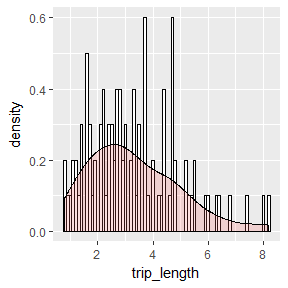Once the population of anglers has been created, the next function to apply is the get_total_values() function. In get_total_values(), the user specifies the start time of the creel surveyor, the end time of the surveyor, and the wait time of the surveyor. Here is where the user also specifies the sampling probability of the anglers (in most cases, equal to $$\frac{waitTime}{fishingDayLength}$$) and the mean catch rate of the fishery. There are a number of a default settings in the get_total_values() function; see ?get_total_values for a description of how the function handles NULL values for startTime, endTime, and waitTime. startTime and waitTime are the times that the surveyor started and waited at the access point. totalCatch and trueEffort are the total (or real) values for catch and effort. meanLambda is the mean catch rate for all anglers. Even though we assigned meanCatchRate to get_total_values(), individual mean catch rates are simulated by rgamma() with shape equal to meanCatchRate and rate equal to 1.

For this walk through, we’ll schedule the surveyor to work for a total of eight hours at the sole access point in our fishery:


anglers %>%
get_total_values(start_time = 0, wait_time = 8, sampling_prob = 8/12, mean_catch_rate = 2.5)
#>   n_observed_trips total_observed_trip_effort n_completed_trips
#> 1               86                   586.0279                50
#>   total_completed_trip_effort total_completed_trip_catch start_time
#> 1                    222.9294                   530.1671          0
#>   wait_time total_catch true_effort mean_lambda
#> 1         8    795.6116    417.0699     2.39971

get_total_values() returns a single row data frame with several columns. The output of get_total_values() is the catch and effort data observed by the surveyor during their wait at the accss point along with the “true” values for catch and effort. (Obviously, we can’t simulate biological data but, if an agency’s protocol directed the surveyor to collect biological data, that could be analyzed with other R functions.)

In the output from get_total_values(), n_observed_trips is the number of trips that the surveyor observed, including anglers that arrived after she started her day and anglers that were there for the duration of her time at the access point. total_observed_trip_effort is the effort expended by those parties; because the observed trips were not complete, she did not count their catch. n_completed_trips is the number of anglers that completed their trips while she was onsite, total_completed_trip_effort is the effort expended by those anglers, and total_completed_trip_catch is the number of fish caught by those parties. Catch is both the number of fish harvested and those caught and released.

### Estimating catch and effort

Effort and catch are estimated from the Bus Route Estimator:

$\widehat{E} = T\sum\limits_{i=1}^n{\frac{1}{w_{i}}}\sum\limits_{j=1}^m{\frac{e_{ij}}{\pi_{j}}}$

where

• E = estimated total party-hours of effort;
• T = total time to complete a full circuit of the route, including travelling and waiting;
• wi = waiting time at the ith site (where i = 1, …, n sites);

and

• eij = total time that the jth car (or trailer) is parked at the ith site while the agent is at that shite (where j = 1, …, n sites).

Catch rate is calculated from the Ratio of Means equation:

$\widehat{R_1} = \frac{\sum\limits_{i=1}^n{c_i/n}}{\sum\limits_{i=1}^n{L_i/n}}$

where

• ci is the catch for the ith sampling unit

and
* Li is the length of the fishing trip at the tie of the interview.

For incomplete surveys, Li represents an incomplete trip.

simulate_bus_route() calculates effort and catch based upon these equations. See ?simulate_bus_route for references that include a more detailed discussion of these equations.

simulate_bus_route() calls make_anglers() and get_total_values() so many of the same arguments we passed in the previous functions will need to be passed to simulate_bus_route(). The new arguent, nsites, is the number of sites visited by the surveyor. In more advanced simulations (see the examples in ?simulate_bus_route), you can pass strings of values for startTime, waitTime, nsites, and nanglers to simulate a bus route-type survey rather than just a single access-point survey.


sim <- simulate_bus_route(start_time = 0, wait_time = 8, n_sites = 1, n_anglers = 100,
sampling_prob = 8/12, mean_catch_rate = 2.5, fishing_day_length = 12)

sim
#>       Ehat catch_rate_ROM true_catch true_effort mean_lambda
#> 1 585.9488       2.607587   866.5934    403.0374    2.481576

The output from simulate_bus_route() is a dataframe with values for Ehat, catchRateROM (the ratio of means catch rate), trueCatch, trueEffort, and meanLambda. Ehat is the estimated total effort from the Bus Route Estimator above and catchRateROM is catch rate estimated from the Ratio of Means equation. trueCatch, trueEffort, and meanLambda are the same as before. Multiplying Ehat by catchRateROM gives an estimate of total catch: 1527.9124526.

### Conducting multiple simulations

With information about the fishery, the start and wait times of the surveyor, the sampling probability, mean catch rate, and fishing day length, we can run multiple simulations with conduct_multiple_surveys(). conduct_multiple_surveys() is a wrapper that calls the other three functions in turn and compiles the values into a data frame for easy plotting or analysis. The only additional argument needed is the nsims value which tells the function how many simulations to conduct. For the sake of this simple simulation, let’s assume that the creel survery works five days a week for four weeks (i.e. 20 days):


sim <- conduct_multiple_surveys(n_sims = 20, start_time = 0, wait_time = 8, n_sites = 1,
n_anglers = 100, sampling_prob = 8/12,
mean_catch_rate = 2.5, fishing_day_length = 12)

sim
#>        Ehat catch_rate_ROM true_catch true_effort mean_lambda
#> 1  582.5113       2.197079   744.9890    408.1091    2.238827
#> 2  525.6581       2.610515   962.0484    371.0789    2.788176
#> 3  532.4813       2.593379   774.0897    376.5876    2.364251
#> 4  636.9765       2.069540   776.0824    444.2898    2.275464
#> 5  551.9909       2.255368   816.2178    392.7341    2.518792
#> 6  666.7207       2.161836   799.9225    461.0866    2.379170
#> 7  571.7152       2.399565   817.8163    402.0583    2.547475
#> 8  621.4893       2.069414   731.0183    441.1592    2.134023
#> 9  608.1691       2.328550   854.8820    426.5282    2.305846
#> 10 531.5312       2.260759   764.4231    380.1294    2.258321
#> 11 600.5033       2.910176   844.1526    412.9629    2.757026
#> 12 583.2813       2.542058   836.5646    401.1198    2.613398
#> 13 621.7530       2.526983   886.7834    422.6602    2.482501
#> 14 614.4349       2.658935   782.2079    422.5720    2.459972
#> 15 593.8837       2.748767   936.2094    411.7710    2.764624
#> 16 579.6193       2.344677   833.4941    410.2437    2.377972
#> 17 610.3543       2.395208   911.6421    429.0311    2.658027
#> 18 557.2910       2.300684   862.2922    396.4331    2.498155
#> 19 566.0941       2.073266   729.5827    396.8897    2.138644
#> 20 608.2846       2.128812   731.1389    432.0415    1.974324

With the output from multiple simulations, an analyst can evaluate how closely the creel survey they’ve designed mirrors reality. A lm() of estimated catch as a function of trueCatch can tell us if the survey will over or under estimate reality:


mod <-
sim %>%
lm((Ehat * catch_rate_ROM) ~ true_catch, data = .)

summary(mod)
#>
#> Call:
#> lm(formula = (Ehat * catch_rate_ROM) ~ true_catch, data = .)
#>
#> Residuals:
#>     Min      1Q  Median      3Q     Max
#> -185.54  -71.15  -22.28   66.09  322.50
#>
#> Coefficients:
#>             Estimate Std. Error t value Pr(>|t|)
#> (Intercept) 474.8766   376.0562   1.263   0.2228
#> true_catch    1.1256     0.4572   2.462   0.0241 *
#> ---
#> Signif. codes:  0 '***' 0.001 '**' 0.01 '*' 0.05 '.' 0.1 ' ' 1
#>
#> Residual standard error: 136.5 on 18 degrees of freedom
#> Multiple R-squared:  0.2519, Adjusted R-squared:  0.2103
#> F-statistic: 6.061 on 1 and 18 DF,  p-value: 0.02414

Plotting the data and the model provide a good visual means of evaluating how close our estimates are to reality:


#Create a new vector of the estimated effort multiplied by estimated catch rate
sim <-
sim %>%
mutate(est_catch = Ehat * catch_rate_ROM)

sim %>%
ggplot(aes(x = true_catch, y = est_catch)) +
geom_point() +
geom_abline(intercept = mod$coefficients, slope = mod$coefficients,
colour = "red", size = 1.01)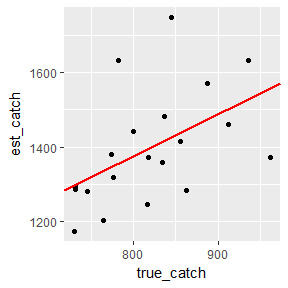The closer the slope parameter estimate is to 1 and the intercept parameter estimate is to 0, the closer our estimate of catch is to reality.

We can create a model and plot of our effort estimates, too:


mod <-
sim %>%
lm(Ehat ~ true_effort, data = .)

summary(mod)
#>
#> Call:
#> lm(formula = Ehat ~ true_effort, data = .)
#>
#> Residuals:
#>      Min       1Q   Median       3Q      Max
#> -12.0376  -6.0464  -0.9879   3.1929  16.9332
#>
#> Coefficients:
#>              Estimate Std. Error t value Pr(>|t|)
#> (Intercept) -51.07503   32.32989   -1.58    0.132
#> true_effort   1.55183    0.07835   19.80 1.14e-13 ***
#> ---
#> Signif. codes:  0 '***' 0.001 '**' 0.01 '*' 0.05 '.' 0.1 ' ' 1
#>
#> Residual standard error: 8.011 on 18 degrees of freedom
#> Multiple R-squared:  0.9561, Adjusted R-squared:  0.9537
#> F-statistic: 392.2 on 1 and 18 DF,  p-value: 1.141e-13

#Create a new vector of the estimated effort multiplied by estimated catch rate

sim %>%
ggplot(aes(x = true_effort, y = Ehat)) +
geom_point() +
geom_abline(intercept = mod$coefficients, slope = mod$coefficients,
colour = "red", size = 1.01)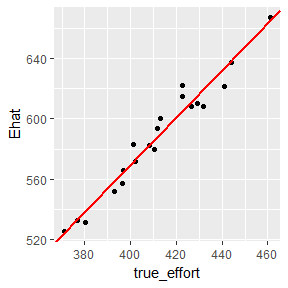### Can we observe ALL trips?

If the start and wait time equals 0 and the length of the fishing day, respectively, the creel surveyor can observe all completed trips, though she’d likely be unhappy having to work 12 hours. The inputs have to be adjusted to allow her to arrive at time 0, stay for all 12 hours, and have a probability of 1.0 at catching everyone:


start_time <- 0
wait_time <- 12
sampling_prob <- 1

sim <- conduct_multiple_surveys(n_sims = 20, start_time = start_time, wait_time = wait_time,
n_sites = 1, n_anglers = 100, sampling_prob = 1,
mean_catch_rate = 2.5, fishing_day_length = 12)

sim
#>        Ehat catch_rate_ROM true_catch true_effort mean_lambda
#> 1  768.7004       2.470043   824.7229    768.7004    2.511069
#> 2  708.7170       2.599554   806.7401    708.7170    2.495052
#> 3  759.9584       2.416612   854.5432    759.9584    2.358045
#> 4  783.3415       2.715485   892.3400    783.3415    2.663605
#> 5  728.9695       2.126378   691.4138    728.9695    2.128315
#> 6  724.7953       2.183654   724.3334    724.7953    2.273502
#> 7  695.8944       2.599302   844.9681    695.8944    2.614189
#> 8  789.6448       2.410761   814.9992    789.6448    2.467584
#> 9  781.7185       2.672259   876.0535    781.7185    2.706500
#> 10 753.8212       2.370904   822.9531    753.8212    2.410414
#> 11 814.7701       2.519984   879.0222    814.7701    2.583332
#> 12 811.6684       2.305741   759.6655    811.6684    2.385969
#> 13 751.8314       2.374681   773.6552    751.8314    2.429269
#> 14 753.1468       2.419120   836.7430    753.1468    2.436932
#> 15 776.5254       2.162192   817.4036    776.5254    2.237419
#> 16 738.9103       2.666906   885.2465    738.9103    2.609013
#> 17 784.0078       2.284436   807.6391    784.0078    2.316637
#> 18 784.3040       2.603432   874.8825    784.3040    2.618557
#> 19 802.6489       2.429314   860.2270    802.6489    2.333058
#> 20 774.0712       2.567579   905.7421    774.0712    2.469119
#> Warning in summary.lm(mod): essentially perfect fit: summary may be
#> unreliable
#>
#> Call:
#> lm(formula = Ehat ~ true_effort, data = .)
#>
#> Residuals:
#>        Min         1Q     Median         3Q        Max
#> -1.008e-13 -9.010e-16  1.612e-14  2.442e-14  1.043e-13
#>
#> Coefficients:
#>              Estimate Std. Error   t value Pr(>|t|)
#> (Intercept) 2.034e-13  2.840e-13 7.160e-01    0.483
#> true_effort 1.000e+00  3.712e-16 2.694e+15   <2e-16 ***
#> ---
#> Signif. codes:  0 '***' 0.001 '**' 0.01 '*' 0.05 '.' 0.1 ' ' 1
#>
#> Residual standard error: 5.282e-14 on 18 degrees of freedom
#> Multiple R-squared:      1,  Adjusted R-squared:      1
#> F-statistic: 7.258e+30 on 1 and 18 DF,  p-value: < 2.2e-16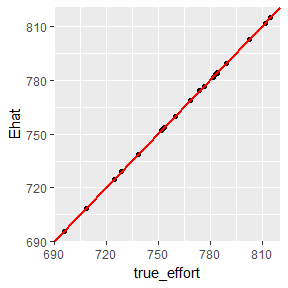### Another simulation

If our hypothetical fishery suddenly gained another access point and the original 100 anglers were split between the two access points equally, what kind of information would a creel survey capture? We could ask our surveyor to split her eight-hour work day between both access points, but she’ll have to drive for 0.5 hours to get from one to another. Of course, that 0.5 hour of drive time will be a part of her work day so she’ll effectively have 7.5 hours to spend at access points counting anglers and collecting data.


start_time <- c(0, 4.5)
wait_time <- c(4, 3.5)
n_sites = 2
n_anglers <- c(50, 50)
fishing_day_length <- 12
sampling_prob <- sum(wait_time)/fishing_day_length

sim <- conduct_multiple_surveys(n_sims = 20, start_time = start_time, wait_time = wait_time,
n_sites = n_sites, n_anglers = n_anglers,
sampling_prob = sampling_prob, mean_catch_rate = 2.5,
fishing_day_length = fishing_day_length)

sim
#>        Ehat catch_rate_ROM true_catch true_effort mean_lambda
#> 1  336.3974       2.446137   842.4591    194.2340    2.501437
#> 2  459.4682       2.461493   794.7933    216.4546    2.514877
#> 3  363.0755       2.157886   760.6865    233.0647    2.359580
#> 4  422.7744       2.310826   787.1008    223.0891    2.374984
#> 5  413.9636       2.549926   906.0712    222.9362    2.577263
#> 6  409.6488       2.585385   743.7690    217.4829    2.351497
#> 7  429.4646       2.806555   889.1171    245.4681    2.555515
#> 8  437.3585       2.494798   869.9422    237.6559    2.438136
#> 9  418.4751       3.389806   927.2633    225.8867    2.674165
#> 10 435.0700       2.468669   836.1957    246.1885    2.289779
#> 11 508.9174       2.480358   840.6856    247.3459    2.325625
#> 12 401.5740       2.808633   922.8538    218.0649    2.880003
#> 13 380.6494       3.202355   953.2290    230.1477    2.686220
#> 14 411.1600       2.460808   957.5743    214.7786    2.713318
#> 15 438.6053       2.880876   742.9910    224.8079    2.279269
#> 16 501.4253       1.962584   753.3787    231.2408    2.422737
#> 17 482.2694       2.375788   803.4919    234.8892    2.316639
#> 18 502.1387       2.132380   843.9224    234.5807    2.393269
#> 19 420.0203       2.400470   734.3253    223.6434    2.292050
#> 20 492.1539       2.721672   858.8633    215.6486    2.493468
#>
#> Call:
#> lm(formula = Ehat ~ true_effort, data = .)
#>
#> Residuals:
#>     Min      1Q  Median      3Q     Max
#> -80.775 -20.218  -7.547  36.599  78.212
#>
#> Coefficients:
#>             Estimate Std. Error t value Pr(>|t|)
#> (Intercept)  43.6023   173.5401   0.251   0.8045
#> true_effort   1.7173     0.7637   2.249   0.0373 *
#> ---
#> Signif. codes:  0 '***' 0.001 '**' 0.01 '*' 0.05 '.' 0.1 ' ' 1
#>
#> Residual standard error: 42.55 on 18 degrees of freedom
#> Multiple R-squared:  0.2193, Adjusted R-squared:  0.1759
#> F-statistic: 5.056 on 1 and 18 DF,  p-value: 0.0373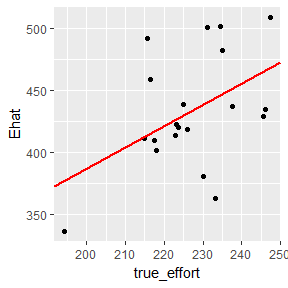### Even more simulations

Ultimately, the creel survey simulation can be as complicated as a creel survey. If a survey requires multiple clerks, several simulations can be coupled together to act as multiple surveryors. To accomodate weekends or holidays (i.e., increased angler pressure), additional simulations with different wait times and more anglers (to simulate higher pressure) can be built into the simulation. For example, if we know that angler pressure is 50% higher at the two access points on weekends, we can hire a second clerk to sample 8 hours a day on the weekends–one day at each access point–and add the weekend data to the weekday data.


#Weekend clerks
start_time_w <- 2
wait_time_w <- 10
n_sites <- 1
n_anglers_w <- 75
fishing_day_length <- 12
sampling_prob <- 8/12

sim_w <- conduct_multiple_surveys(n_sims = 8, start_time = start_time_w,
wait_time = wait_time_w, n_sites = n_sites,
n_anglers = n_anglers_w, sampling_prob = sampling_prob,
mean_catch_rate = 2.5, fishing_day_length = fishing_day_length)

sim_w
#>       Ehat catch_rate_ROM true_catch true_effort mean_lambda
#> 1 654.4297       2.405069   636.0419    437.8568    2.514499
#> 2 635.3440       2.498353   602.0437    423.5627    2.453219
#> 3 711.8827       2.314211   618.1801    474.5885    2.379199
#> 4 596.5517       2.349445   590.9965    398.3901    2.403765
#> 5 683.4622       2.632500   697.3532    457.2936    2.603457
#> 6 649.8841       2.303446   573.7912    434.3805    2.387601
#> 7 589.8644       2.657115   752.9906    394.7195    2.607380
#> 8 647.9023       2.553308   651.7656    431.9349    2.489279

#Add the weekday survey and weekend surveys to the same data frame
mon_survey <-
sim_w %>%
bind_rows(sim)

mod <-
mon_survey %>%
lm(Ehat ~ true_effort, data = .)

summary(mod)
#>
#> Call:
#> lm(formula = Ehat ~ true_effort, data = .)
#>
#> Residuals:
#>     Min      1Q  Median      3Q     Max
#> -75.643 -17.224  -5.803  10.862  71.865
#>
#> Coefficients:
#>              Estimate Std. Error t value Pr(>|t|)
#> (Intercept) 192.08255   22.16532   8.666 3.82e-09 ***
#> true_effort   1.05823    0.07377  14.345 7.29e-14 ***
#> ---
#> Signif. codes:  0 '***' 0.001 '**' 0.01 '*' 0.05 '.' 0.1 ' ' 1
#>
#> Residual standard error: 36.71 on 26 degrees of freedom
#> Multiple R-squared:  0.8878, Adjusted R-squared:  0.8835
#> F-statistic: 205.8 on 1 and 26 DF,  p-value: 7.291e-14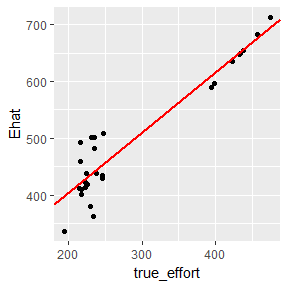Hopefully, this vignette has shown you how to build and simulate your own creel survey. It’s flexible enough to estimate monthly or seasonal changes in fishing day length, changes in the mean catch rate, increased angler pressure on weekends, and any number of access sites, start times, wait times, and sampling probabilities. The output from conduct_multiple_surveys() allows the user to estiate variability in the catch and effort estimates (e.g., relative standard error) to evaluate the most efficient creel survey for their fishery.